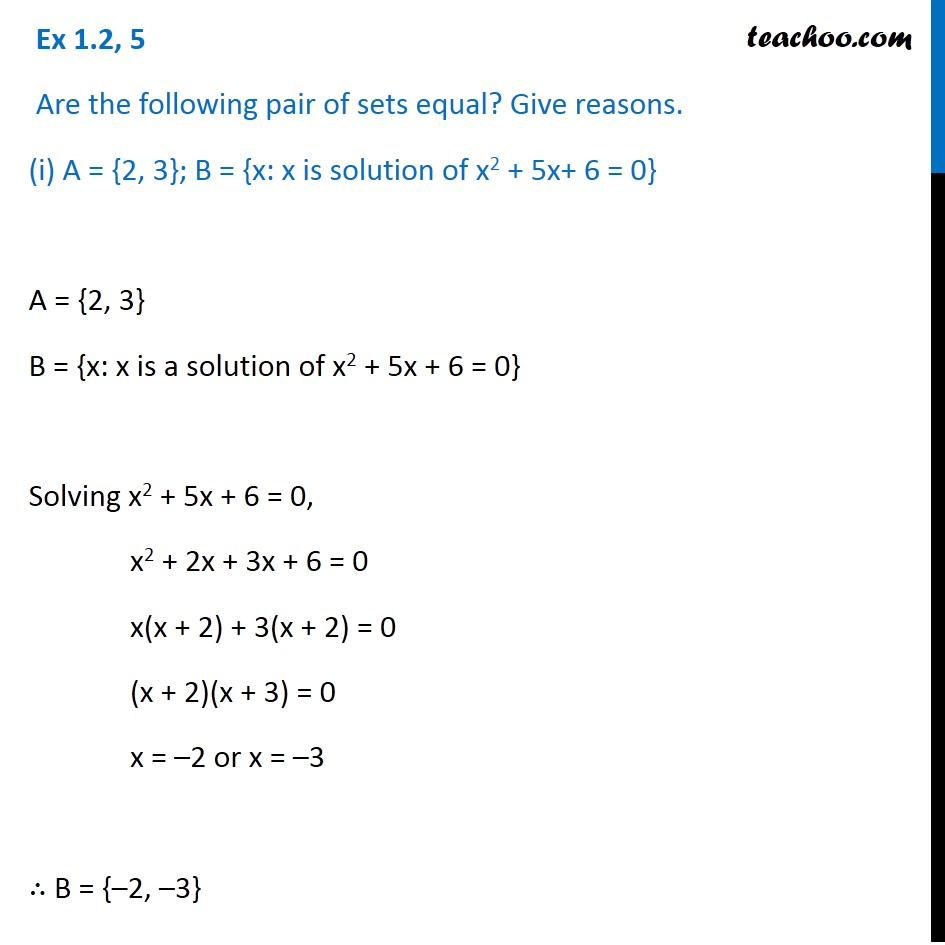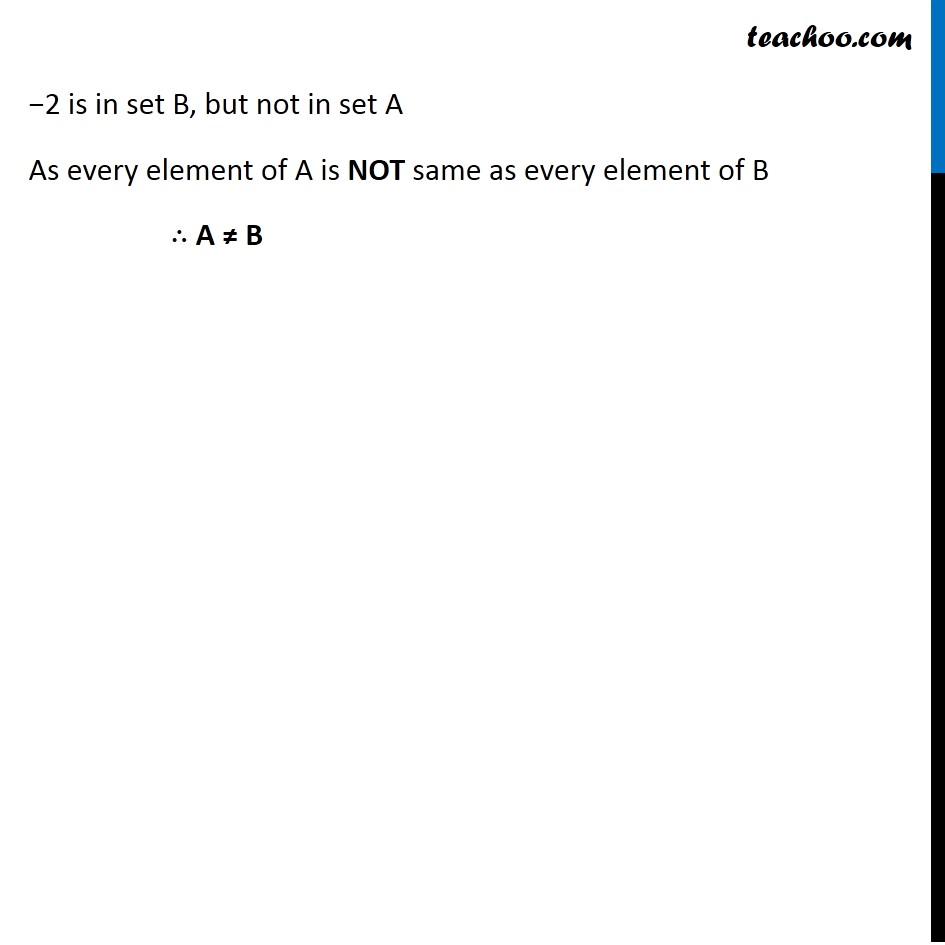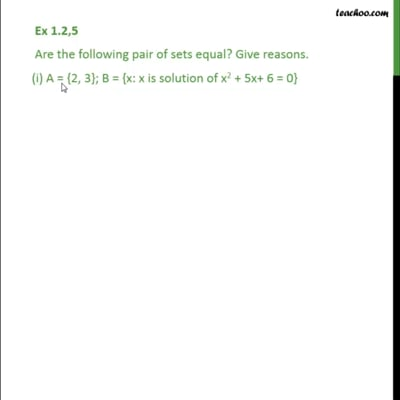Equal sets

Chapter 1 Class 11 Sets
Concept wiseThis video is only available for Teachoo black users

Introducing your new favourite teacher - Teachoo Black, at only ₹83 per month

### Transcript

Ex 1.2, 5 Are the following pair of sets equal? Give reasons. (i) A = {2, 3}; B = {x: x is solution of x2 + 5x+ 6 = 0} A = {2, 3} B = {x: x is a solution of x2 + 5x + 6 = 0} Solving x2 + 5x + 6 = 0, x2 + 2x + 3x + 6 = 0 x(x + 2) + 3(x + 2) = 0 (x + 2)(x + 3) = 0 x = –2 or x = –3 ∴ B = {–2, –3} −2 is in set B, but not in set A As every element of A is NOT same as every element of B ∴ A ≠ B